# The double pendulum

Here, we analyze the motions of a planar double pendulum, such as the one illustrated in Figure 1 below. The double pendulum is a fascinating system to examine because of the richness of its chaotic dynamic behavior.

Contents

## Equations of motion

Referring to Figure 1, the planar double pendulum we consider consists of two pendula that swing freely in the vertical plane and are connected to each other by a smooth pin joint, where each pendulum comprises a light rigid rod with a concentrated mass on one end. The first pendulum, whose other end pivots without friction about the fixed origin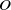, has length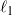and mass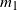, while the second pendulum’s length and mass are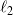and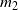, respectively. The dynamic behavior of the double pendulum is captured by the angles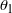and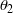that the first and second pendula, respectively, make with the vertical, where both pendula are hanging vertically downward when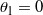and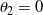. Consequently, the rotations of the pendula are characterized by the rotation tensors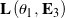and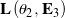.

We can obtain the equations of motion for the double pendulum by applying balances of linear and angular momenta to each pendulum’s concentrated mass or, equivalently, by employing Lagrange’s equations of motion in the form

(1)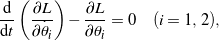where the Lagrangian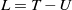depends on the double pendulum’s kinetic energy

(2)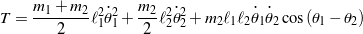and its potential energy

(3)Evaluating (1) and then introducing the dimensionless mass, length, and time parameters

(4)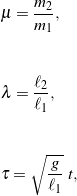respectively, yields the following pair of nondimensional, second-order, ordinary differential equations governing the double pendulum’s behavior:

(5)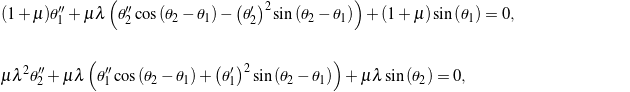where the prime symbol denotes differentiation with respect to the dimensionless time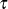. If we arrange the anglesandin a column vector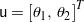, then the system of differential equations in (5) can be written in the convenient second-order matrix-vector form, where

(6)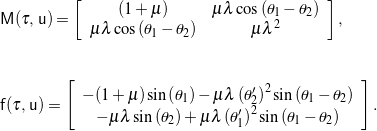Before we can numerically integrate the double pendulum’s equations of motion in MATLAB, we must express the equations in first-order form. To do so, we introduce the state vector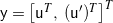such that

(7)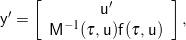which is a form of the equations of motion that is suitable for numerical integration in MATLAB.

## Simulation and animation

Provided a set of initial conditions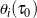and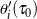, we may now numerically compute the evolution of each pendulum’s angular displacement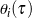and then construct the motion of the overall double pendulum. Figure 2 contains the animated behavior of the double pendulum for one such simulation. In this case, the pendulum was dragged into the initial configuration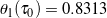andand then released from rest: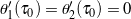. The parameter values were taken as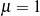and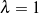, i.e., the masses and lengths of the pendula were identical:and. Also shown in Figure 2 is an animation of the double pendulum’s single-particle representation moving on its so-called configuration manifold, which in this case is a torus parameterized by the angular displacementsand. Casey showed that the response of a system of particles (such as the idealized double pendulum we consider here) can be reduced to the motion of a single representative particle moving on a manifold; we refer the interested reader to Casey’s paper  for the details of this single-particle construction. Finally, note that all motions of the double pendulum, regardless of their initial conditions, must conserve the total mechanical energy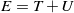because the pendulum swings freely with no dissipation.